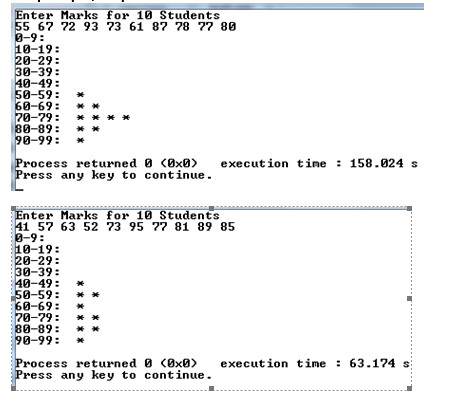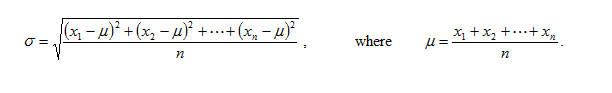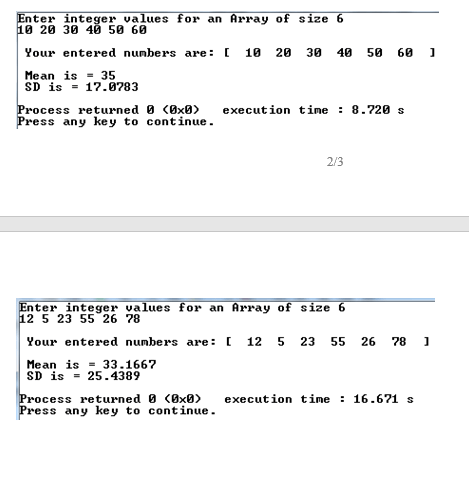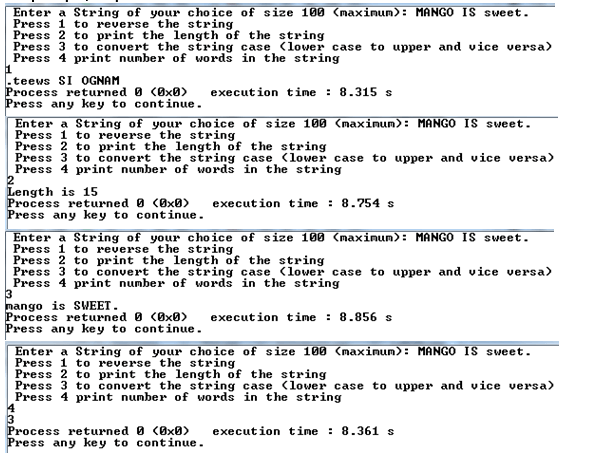# Homework #3 Solved

30.00 \$ 15.00 \$

Category:

You 'll get a: . zip file

## Description

Write a program that prompts the user to enter grades of TEN students. The program then distributes the grades in different ranges (i.e. 0-9, 10-19, 20-29, 30-39, 40-49, 50-59, 60-69, 70-79, 80-89, 90-99) and prints a star (‘*’) in front of each category for each occurrence of the grade falling the corresponding range, as shown in the output given below.  Sample Input/Output:Exercise #2: Mean and Standard Deviation

Write a program that prompts the user to enter integer values for the array of size N (in this case N is 6). The program then calculates mean and standard deviation for the data entered.

Hints: The standard deviation s of a finite data set x1, x2, …, xn is defined by the following formula, µ is the mean:Sample Input/Output:Exercise #3: Some operations on Strings

Write a program that uses a C-string (character array) to store a string entered from the keyboard. The program will then provide 4 options about operations performed on the input string in the form of a menu, as shown in the following sample Input/Output. The 4 operations are given below:1.       reverse the string2.       print the length of the string3.       convert the string case, i.e. from upper case to lower and from lower to upper case4.       print number of words in the string Note: It is not allowed to use the string library functions for the 4 operations. Sample Input/Output: